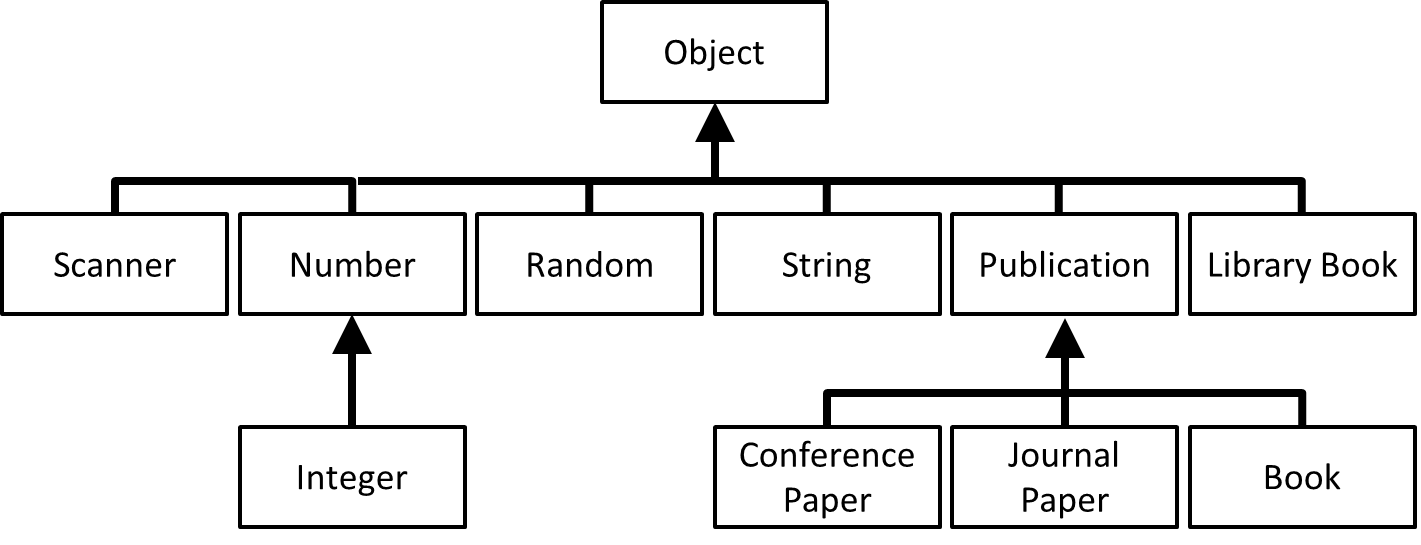# Part of the class hierarchy# The `Object` class

• There is one class, `Object`, that every other class is a direct or indirect subclass of
• So, when there is no explicit `extends` clause, implicitly you are saying `extends Object`
• When you are defining your own classes, you need to consider how objects will be given a string representation, compared for equality, given a hash code, copied and disposed of
• `Object` already contains default definitions for all these, which will be inherited
• However, the defaults often don't do what you want, and so will need to be overridden

# `toString`: the true story

• `print` and `println` automatically call `toString`
• Every class has a definition of `toString` because...
• `Object` includes a `toString` method, which will be inherited
• But this version of `toString` simply causes the reference ('pointer') to be printed
• So we override it with a definition that returns a more meaningful string
• E.g. add to `Dog`:
```public String toString() {
return name + " is a " + age + " year old " + breed;
}```

# Equality testing: the true story

• `==` compares references ('pointers')
• to do something other than this, use a method, e.g. one called `equals`
• Every class has a definition of `equals` because...
• `Object` includes an `equals` method, which will be inherited
• But this version of `equals` simply compares 'pointers' (i.e. it does the same as `==`)
• So we override it with a definition that does a more meaningful comparison
• E.g. add to `Doog`:
```public boolean equals(Object obj) {
if (this == obj) {
return true;
} else if (obj == null || getClass() != obj.getClass()) {
return false;
}
Dog d = (Dog) obj;
return (name.equals(d.name) && age == d.age && breed.equals(d.breed));
}```

# Summary

Primitive typesReference types
Examples
`int`, `double`, `boolean` arrays, `String`, `Scanner`, `Dog`
Contents
Contains a value Contains `null` or a reference ('pointer') to an object
`System.out.println(...)`
Displays the value Runs `toString`
• `toString` inherited from `Object` displays the pointer
• override `toString` to display something more meaningful
Assignment
Copies the value Copies the reference (hence two pointers to the same object)
Equality testing
`==` tests for equal values
• `==` tests for equal references (pointing to the same object
• `equals`, inherited from `Object`, is like `==`
• override `equals` to compare the objects' instance variables
Passing a parameter to a method
Copies the value, so changes made in the method do not affect the original Copies the reference (hence two pointers to the same object), so changes to the object made in the method do affect the original

# Polymorphic data structures, again

• For real generality, we can define data structures that contain objects of class `Object`
• can put any kind of object into them, including mixtures
• E.g. an array of `Object`s:
```Object[] a = new Object;
a = new Dog("Charles", 2, "Charlywawa");
a = new Dog("Colin", 7, "Colombian Drug Lord");
a = new Cow();```
• We can't put values of type `int` and the other primitive types into these data structures because they are not objects
• This is why we have wrapper classes like `Integer`, `Double` and `Boolean` – to turn primitives into objects:
`a = new Integer(25);`
• A nice feature is autoboxing (and unboxing), which means the following automatically uses the wrapper class:
`a = 25;`

# A complication

• Suppose we have a polymorphic array of `Object`s but that we have only been inserting `LibraryBook`s into the array, e.g.:
```Object[] library = new Object;
library = new LibraryBook(...);
library = new LibraryBook(...);
library = new LibraryBook(...);
// etc.```
• We know that we have only inserted `LibraryBook`s so the only objects we can 'take out' of the array are `LibraryBook`s, but Java won't trust us
• The Java compiler will not be happy with this:
```LibraryBook b = library; // error here
b.acquire();```
nor with this:
```Object b = library;
b.acquire(); // error here```

# The solution: casting

• To persuade the Java compiler that it is OK for us to store the object (which we know is a `LibraryBoook`) in the variable `b`, we must cast:
```LibraryBook b = (LibraryBook) library;
b.acquire();```
Or to persuade it that it is OK to apply one of `LibraryBook`s methods, we must cast:
```Object b = library;
((LibraryBook) b).acquire();```
• By casting, we are promising that what comes out of the array will in fact be a `LibraryBook`
• There's another example of casting in the definition of `equals` earlier
Q: Why was it needed?

# Run-time type errors

• So now what happens if the array is not exclusively `LibraryBook`s? (We lied to the compiler)
```Object[] library = new Object;
library = new LibraryBook(...);
library = new LibraryBook(...);
library = new Cow( );
LibraryBook b = (LibraryBook) library;
b.acquire();```
• We fooled the compiler: no compile-time error
• But we get a run-time error (class cast exception)
• So don't lie!
• This is why Java is mostly statically-typed: some type errors can only be discovered at run-time, when a cast fails